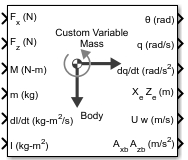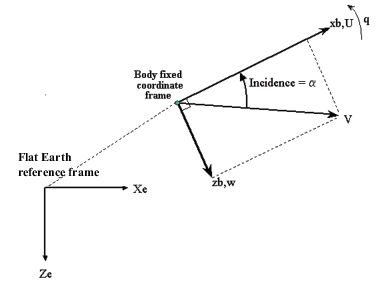Main Content

# Custom Variable Mass 3DOF (Body Axes)

Implement three-degrees-of-freedom equations of motion of custom variable mass with respect to body axes

• Library:
• Aerospace Blockset / Equations of Motion / 3DOF

•## Description

The Custom Variable Mass 3DOF (Body Axes) block implements three-degrees-of-freedom equations of motion of custom variable mass with respect to body axes. It considers the rotation in the vertical plane of a body-fixed coordinate frame about a flat Earth reference frame. For more information about the rotation and equations of motion, see Algorithms.

## Ports

### Input

expand all

Applied force along the body x-axis, specified as a scalar, in the units selected in Units.

Data Types: `double`

Applied force along the body z-axis, specified as a scalar.

Data Types: `double`

Applied pitch moment, specified as a scalar.

Data Types: `double`

One or more rates of change of mass (positive if accreted, negative if ablated), specified as a scalar.

Data Types: `double`

Mass, specified as a scalar.

Data Types: `double`

Rate of change of inertia tensor, Iyy, specified as scalar.

#### Dependencies

To enable this port, set Mass type to `Custom Variable`.

Data Types: `double`

Inertia tensor, specified as a scalar.

#### Dependencies

To enable this port, set Mass type to ```Custom Variable```.

Data Types: `double`

Gravity, specified as a scalar.

#### Dependencies

To enable this port, set Gravity source to `External`.

Data Types: `double`

Sum of one or more relative velocities at which mass is accreted to or ablated from the body in body-fixed axes, specified as a two-element vector.

#### Dependencies

To enable this port, select Include mass flow relative velocity.

Data Types: `double`

### Output

expand all

Pitch attitude, within ±pi, returned as a scalar, in radians (θ).

Data Types: `double`

Pitch angular rate, specified as a scalar, in radians per second.

Data Types: `double`

Pitch angular acceleration, returned as a scalar, in radians per second squared.

Data Types: `double`

Location of the body in the flat Earth reference frame, (Xe, Ze), returned as a two-element vector.

Data Types: `double`

Velocity of the body resolved into the body-fixed coordinate frame, (u, w), returned as a two-element vector.

Data Types: `double`

Acceleration of the body with respect to the body-fixed coordinate frame, (Ax, Az), returned as a two-element vector.

Data Types: `double`

Accelerations of the body with respect to the inertial (flat Earth) coordinate frame, returned as a two-element vector. You typically connect this signal to the accelerometer.

#### Dependencies

To enable this port, select the Include inertial acceleration check box.

Data Types: `double`

## Parameters

expand all

### Main

Input and output units, specified as `Metric (MKS)`, `English (Velocity in ft/s)`, or `English (Velocity in kts)`.

UnitsForcesMomentAccelerationVelocityPositionMassInertia
`Metric (MKS)` NewtonNewton-meterMeters per second squaredMeters per secondMetersKilogramKilogram meter squared
`English (Velocity in ft/s)` PoundFoot-poundFeet per second squaredFeet per secondFeetSlugSlug foot squared
`English (Velocity in kts)` PoundFoot-poundFeet per second squaredKnotsFeetSlugSlug foot squared

#### Programmatic Use

 Block Parameter: `units` Type: character vector Values: `Metric (MKS)` | `English (Velocity in ft/s)` | `English (Velocity in kts)` Default: `Metric (MKS)`

Mass type, specified according to the following table.

Mass TypeDescriptionDefault for
`Fixed`

Mass is constant throughout the simulation.

`Simple Variable`

Mass and inertia vary linearly as a function of mass rate.

`Custom Variable`

Mass and inertia variations are customizable.

The `Custom Variable` selection conforms to the previously described equations of motion.

#### Programmatic Use

 Block Parameter: `mtype` Type: character vector Values: `Fixed` | `Simple Variable` | `Custom Variable` Default: `'Custom Variable'`

Initial velocity of the body, (V0), specified as a scalar.

#### Programmatic Use

 Block Parameter: `v_ini` Type: character vector Values: `'100'` | scalar Default: `'100'`

Initial pitch attitude of the body, (θ0), specified as a scalar.

#### Programmatic Use

 Block Parameter: `theta_ini` Type: character vector Values: `'0'` | scalar Default: `'0'`

Initial angle between the velocity vector and the body, (α0), specified as a scalar.

#### Programmatic Use

 Block Parameter: `alpha_ini` Type: character vector Values: `'0'` | scalar Default: `'0'`

Initial location of the body in the flat Earth reference frame, specified as a two-element vector.

#### Programmatic Use

 Block Parameter: `pos_ini` Type: character vector Values: `'[0 0]'` | two-element vector Default: `'[0 0]'`

Initial pitch rotation rate, (q0), specified as a scalar.

#### Programmatic Use

 Block Parameter: `q_ini` Type: character vector Values: `'0'` | scalar Default: `'0'`

Gravity source, specified as:

 `External` Variable gravity input to block `Internal` Constant gravity specified in mask

#### Programmatic Use

 Block Parameter: `g_in` Type: character vector Values: `'Internal'` | `'External'` Default: `'Internal'`

Acceleration due to gravity, specified as a double scalar and used if internal gravity source is selected. If gravity is to be neglected in the simulation, this value can be set to `0`.

#### Dependencies

• To enable this parameter, set Gravity Source to `Internal`.

#### Programmatic Use

 Block Parameter: `g` Type: character vector Values: `'9.81'` | scalar Default: `'9.81'`

Select this check box to add a mass flow relative velocity port. This is the relative velocity at which the mass is accreted or ablated.

#### Programmatic Use

 Block Parameter: `vre_flag` Type: character vector Values: `off` | `on` Default: `'off'`

Select this check box to add an inertial acceleration in flat Earth frame output port. You typically connect this signal to the accelerometer.

#### Dependencies

To enable the AxeAze port, select this parameter.

#### Programmatic Use

 Block Parameter: `abi_flag` Type: character vector Values: `'off'` | `'on'` Default: `'off'`

### State Attributes

Assign a unique name to each state. You can use state names instead of block paths during linearization.

• To assign a name to a single state, enter a unique name between quotes, for example, `'velocity'`.

• To assign names to multiple states, enter a comma-separated list surrounded by braces, for example, `{'a', 'b', 'c'}`. Each name must be unique.

• If a parameter is empty (`' '`), no name is assigned.

• The state names apply only to the selected block with the name parameter.

• The number of states must divide evenly among the number of state names.

• You can specify fewer names than states, but you cannot specify more names than states.

For example, you can specify two names in a system with four states. The first name applies to the first two states and the second name to the last two states.

• To assign state names with a variable in the MATLAB® workspace, enter the variable without quotes. A variable can be a character vector, cell array, or structure.

Velocity state names, specified as a comma-separated list surrounded by braces.

#### Programmatic Use

 Block Parameter: `vel_statename` Type: character vector Values: `''` | comma-separated list surrounded by braces Default: `''`

Pitch attitude state name, specified as a character vector.

#### Programmatic Use

 Block Parameter: `theta_statename` Type: character vector Values: `''` | character vector Default: `''`

Position state names, specified as a comma-separated list surrounded by braces.

#### Programmatic Use

 Block Parameter: `pos_statename` Type: character vector Values: `''` | comma-separated list surrounded by braces Default: `''`

Pitch angular rate state name, specified as a character vector.

#### Programmatic Use

 Block Parameter: `q_statename` Type: character vector Values: `''` | scalar Default: `''`

## Algorithms

The Custom Variable Mass 3DOF (Body Axes) block considers the rotation in the vertical plane of a body-fixed coordinate frame about a flat Earth reference frame.The equations of motion are

`$\begin{array}{l}{A}_{xb}=\stackrel{˙}{u}=\frac{{F}_{x}}{m}-\frac{\stackrel{˙}{m}Ur{e}_{b}}{m}-qw-g\mathrm{sin}\theta \\ Vr{e}_{b}={\begin{array}{cc}\left[Ure& Wre\right]\end{array}}_{b}\\ {A}_{yb}=\stackrel{˙}{w}=\frac{{F}_{z}}{m}-\frac{\stackrel{˙}{m}Wr{e}_{b}}{m}+qu+g\mathrm{cos}\theta \\ \stackrel{˙}{q}=\frac{M-{\stackrel{˙}{I}}_{yy}q}{{I}_{yy}}\\ \stackrel{˙}{\theta }=q\\ {A}_{be}=\left[\begin{array}{c}{A}_{xe}\\ {A}_{ze}\end{array}\right]=\frac{{F}_{b}-\stackrel{˙}{m}Ur{e}_{b}}{m}-\overline{g}\\ {A}_{bb}=\left[\begin{array}{c}{A}_{xb}\\ {A}_{yb}\end{array}\right]\end{array}$`

where the applied forces are assumed to act at the center of gravity of the body. Ureb and Wreb are the relative velocities of the mass flow ($\stackrel{˙}{m}$) being added to or ejected from the body in body-fixed axes.

## See Also

Introduced in R2006a

## SupportGet trial now